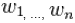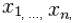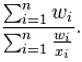Altair® Panopticon

### Weighted Harmonic Mean

The weighted harmonic mean of the selection based on a specified weighting column.

Weighted Harmonic Mean is calculated the same way as the Harmonic Mean. The Harmonic Mean is defined as a special case where all of the weights are equal to 1, and is equivalent to any weighted harmonic mean where all weights are equal.

The formula:

If a set of weightsis associated to the datasetthe weighted harmonic mean is defined bySample 1:

As a simple example, the Weighted Harmonic Mean of 1, 2, and 4 given the weights 5, 6, 7 respectively is:

18/ (5/1 + 6/2 + 7/4) = 18/9.75 = 1.8462